# 1.3   Conditionals and Loops

In the programs that we have examined to this point, each of the statements is executed once, in the order given. Most programs are more complicated because the sequence of statements and the number of times each is executed can vary. We use the term control flow to refer to statement sequencing in a program.

## If statements.

Most computations require different actions for different inputs.

• The following code fragment uses an if statement to put the smaller of two int values in x and the larger of the two values in y, by exchanging the values in the two variables if necessary.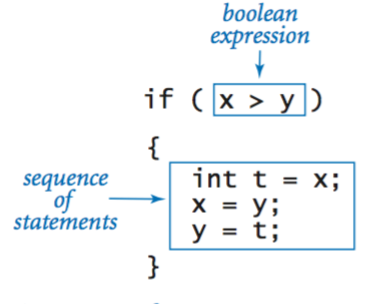• Flip.java uses Math.random() and an if-else statement to print the results of a coin flip.

• The table below summarizes some typical situations where you might need to use an if or if-else statement.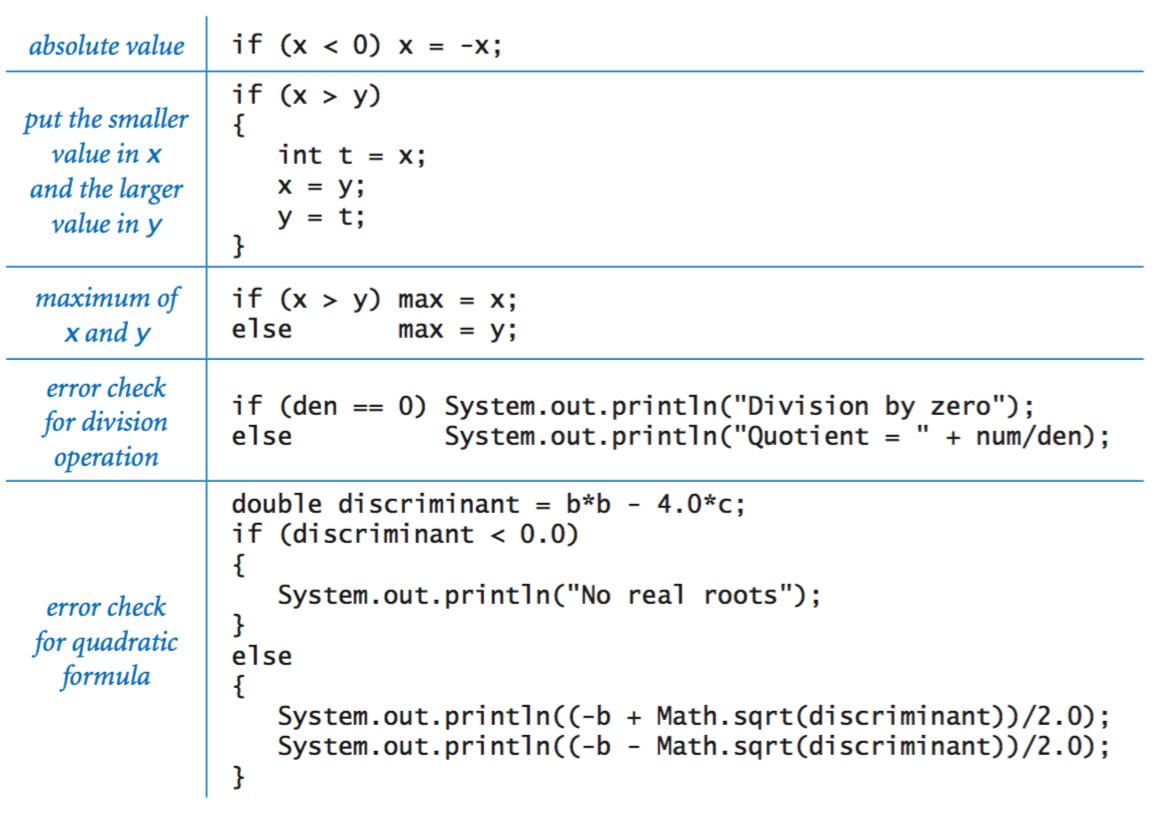## While loops.

Many computations are inherently repetitive. The while loop enables us to execute a group of statements many times. This enables us to express lengthy computations without writing lots of code.
• The following code fragment computes the largest power of 2 that is less than or equal to a given positive integer n.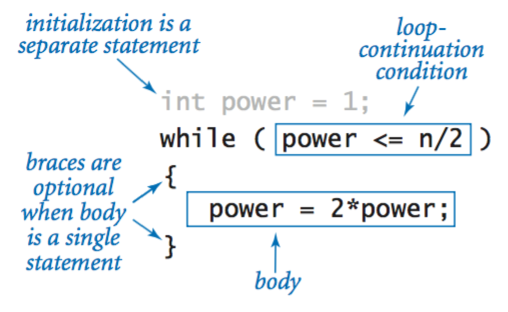• TenHellos.java prints "Hello World" 10 times.
• PowersOfTwo.java takes an integer command-line argument n and prints all of the powers of 2 less than or equal to n.

## For loops.

The for loop is an alternate Java construct that allows us even more flexibility when writing loops.
• For notation. Many loops follow the same basic scheme: initialize an index variable to some value and then use a while loop to test an exit condition involving the index variable, using the last statement in the while loop to modify the index variable. Java's for loop is a direct way to express such loops.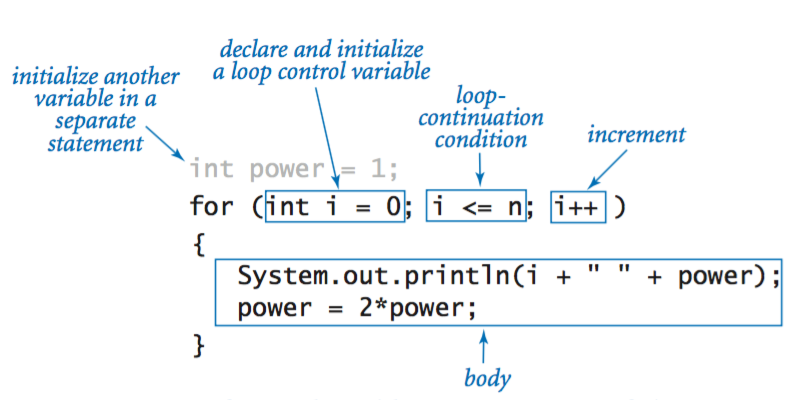• Compound assignment idioms. The idiom i++ is a shorthand notation for i = i + 1.
• Scope. The scope of a variable is the part of the program that can refer to that variable by name. Generally the scope of a variable comprises the statements that follow the declaration in the same block as the declaration. For this purpose, the code in the for loop header is considered to be in the same block as the for loop body.

## Nesting.

The if, while, and for statements have the same status as assignment statements or any other statements in Java; that is, we can use them wherever a statement is called for. In particular, we can use one or more of them in the body of another statement to make compound statements. To emphasize the nesting, we use indentation in the program code.

• DivisorPattern.java has a for loop whose body contains a for loop (whose body is an if-else statement) and a print statement. It prints a pattern of asterisks where the ith row has an asterisk in each position corresponding to divisors of i (the same holds true for the columns).

• MarginalTaxRate.java computes the marginal tax rate for a given income. It uses several nested if-else statements to test from among a number of mutually exclusive possibilities.

## Loop examples.## Applications.

The ability to program with loops and conditionals immediately opens up the world of computation to us.
• Ruler subdivisions. RulerN.java takes an integer command-line argument n and prints the string of ruler subdivision lengths. This program illustrates one of the essential characteristics of loops—the program could hardly be simpler, but it can produce a huge amount of output.
•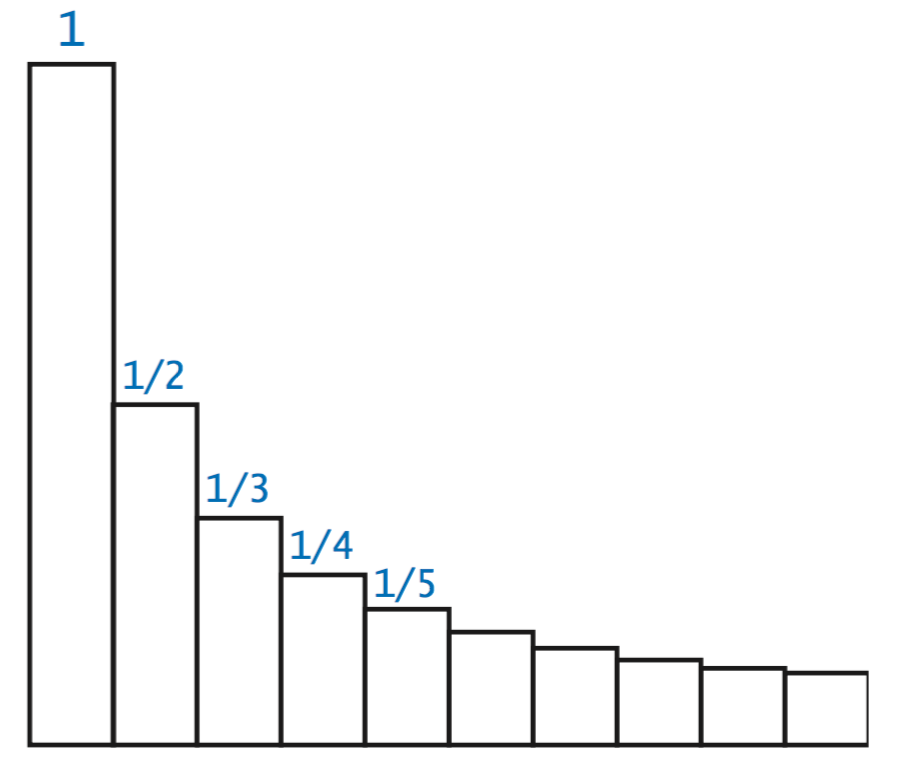• Finite sums. The computational paradigm used in PowersOfTwo.java is one that you will use frequently. It uses two variables—one as an index that controls a loop, and the other to accumulate a computational result. Program HarmonicNumber.java uses the same paradigm to evaluate the sum
$$H_n = \frac{1}{1} + \frac{1}{2} + \frac{1}{3} + \frac{1}{4} + \; \ldots \; + \frac{1}{n}$$

These numbers, which are known as the harmonic numbers, arise frequently in the analysis of algorithms.

• Newton's method.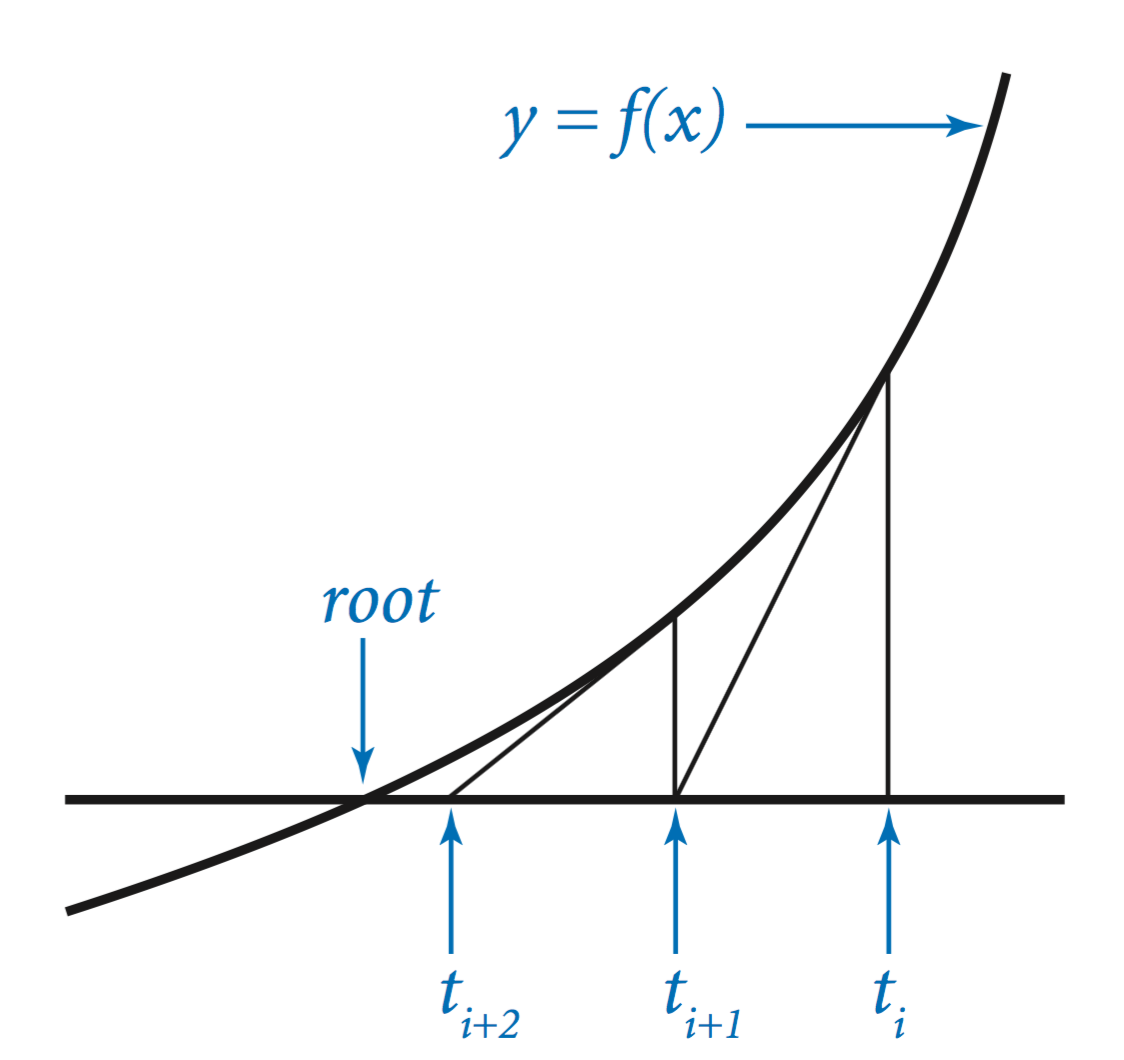Sqrt.java uses a classic iterative technique known as Newton's method to compute the square root of a positive number x: Start with an estimate t. If t is equal to x/t (up to machine precision), then t is equal to a square root of x, so the computation is complete. If not, refine the estimate by replacing t with the average of t and x/t. Each time we perform this update, we get closer to the desired answer.
• Number conversion. Binary.java prints the binary (base 2) representation of the decimal number typed as the command-line argument. It is based on decomposing the number into a sum of powers of 2. For example, the binary representation of 106 is 11010102, which is the same as saying that 106 = 64 + 32 + 8 + 2. To compute the binary representation of n, we consider the powers of 2 less than or equal to n in decreasing order to determine which belong in the binary decomposition (and therefore correspond to a 1 bit in the binary representation).
• Gambler's ruin.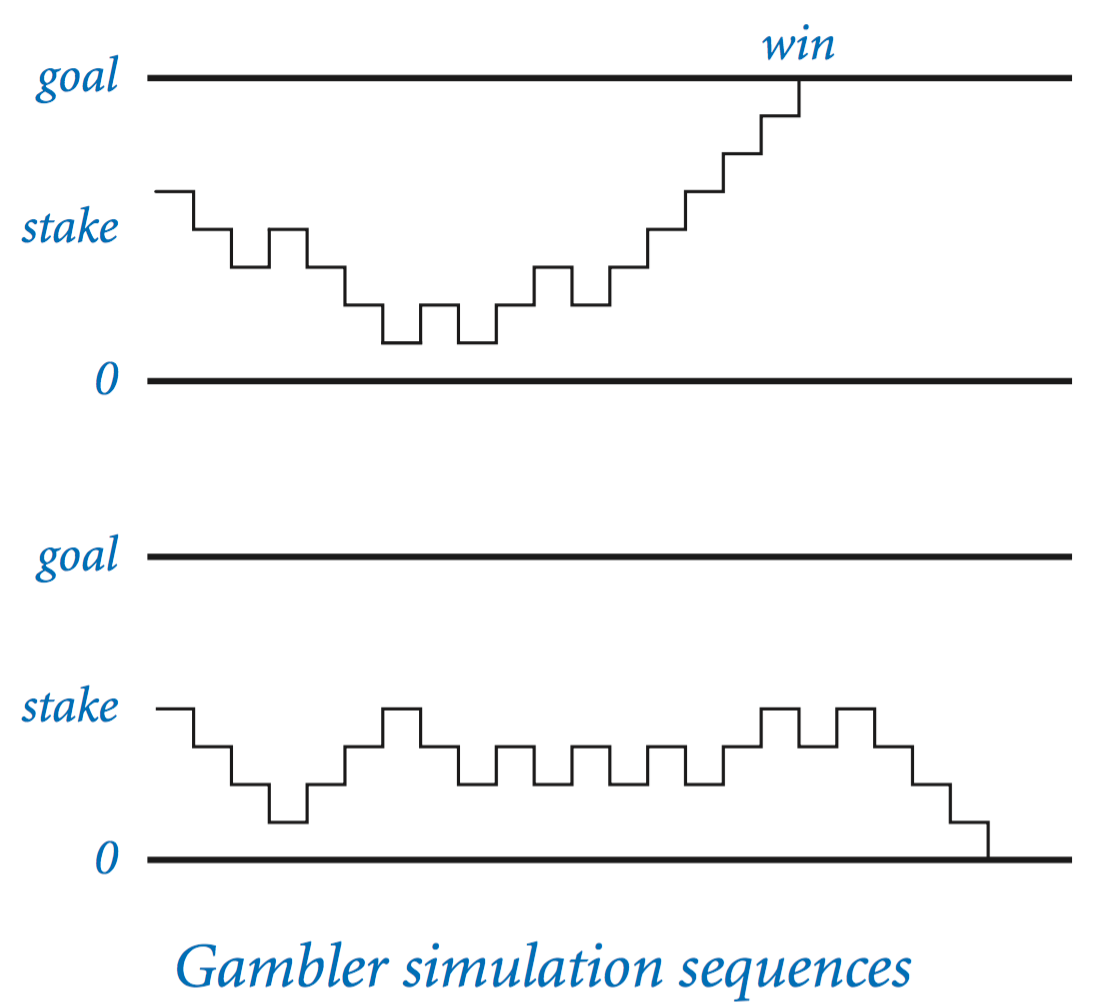Suppose a gambler makes a series of fair $1 bets, starting with$50, and continue to play until she either goes broke or has $250. What are the chances that she will go home with$250, and how many bets might she expect to make before winning or losing? Gambler.java is a simulation that can help answer these questions. It takes three command-line arguments, the initial stake ($50), the goal amount ($250), and the number of times we want to simulate the game.
• Prime factorization. Factors.java takes an integer command-line argument n and prints its prime factorization. In contrast to many of the other programs that we have seen (which we could do in a few minutes with a calculator or pencil and paper), this computation would not be feasible without a computer.

## Other conditional and loop constructs.

To be complete, we consider four more Java constructs related to conditionals and loops. They are used much less frequently than the if, while, and for statements that we've been working with, but it is worthwhile to be aware of them.

• Break statements. In some situations, we want to immediate exit a loop without letting it run to completion. Java provides the break statement for this purpose. Prime.java takes an integer command-line argument n and prints true if n is prime, and false otherwise. There are two different ways to leave this loop: either the break statement is executed (because n is not prime) or the loop-continuation condition is not satisfied (because n is prime).

Note that the break statement does not apply to if or if-else statements. In a famous programming bug, the U.S. telephone network crashed because a programmer intended to use a break statement to exit a complicated if statement.

• Continue statements. Java also provides a way to skip to the next iteration of a loop: the continue statement. When a continue is executed within the body of a for loopy, the flow of control transfers directly to the increment statement for the next iteration of the loop.
• Switch statements. The if and if-else statements allow one or two alternatives. Sometimes, a computation naturally suggests more than two mutually exclusive alternatives. Java provides the switch statement for this purpose. NameOfDay.java takes an integer between 0 and 6 as a command-line argument and uses a switch statement to print the corresponding name of the day (Sunday to Saturday).
• Do–while loops. A do-while loop is almost the same as a while loop except that the loop-continuation condition is omitted the first time through the loop. RandomPointInCircle.java sets x and y so that (x, y) is randomly distributed inside the circle centered at (0, 0) with radius 1.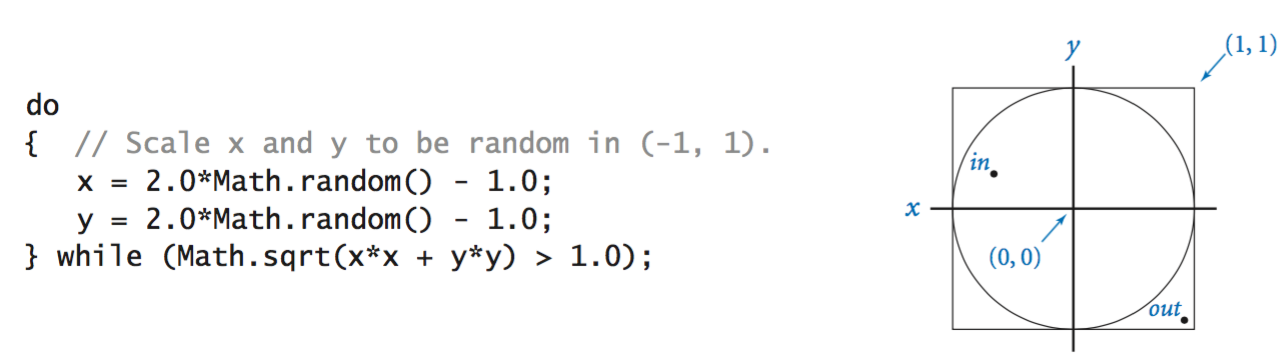With Math.random() we get points that are randomly distributed in the 2-by-2 square center at (0, 0). We just generate points in this region until we find one that lies inside the unit disk. We always want to generate at least one point so a do-while loop is most appropriate. We must declare x and y outside the loop since we will want to access their values after the loop terminates.

We don't use the following two flow control statements in this textbook, but include them here for completeness.
• Conditional operator. The conditional operator ?: is a ternary operator (three operands) that enables you to embed a conditional within an expression. The three operands are separated by the ? and : symbols. If the first operand (a boolean expression) is true, the result has the value of the second expression; otherwise it has the value of the third expression.
 int min = (x < y) ? x : y; 
• Labeled break and continue statements. The break and continue statements apply to the innermost for or while loop. Sometimes we want to jump out of several levels of nested loops. Java provides the labeled break and labeled continue statements to accomplish this. Here is an example.

#### Exercises

1. Write a program AllEqual.java that takes three integer command-line arguments and prints equal if all three are equal, and not equal otherwise.
2. Write a program RollLoadedDie.java that prints the result of rolling a loaded die such that the probability of getting a 1, 2, 3, 4, or 5 is 1/8 and the probability of getting a 6 is 3/8.
3. Rewrite TenHellos.java to make a program Hellos.java that takes the number of lines to print as a command-line argument. You may assume that the argument is less than 1000. Hint: consider using i % 10 and i % 100 to determine whether to use "st", "nd", "rd", or "th" for printing the ith Hello.
4. Write a program FivePerLine.java that, using one for loop and one if statement, prints the integers from 1000 to 2000 with five integers per line. Hint: use the % operator.
5. Write a program FunctionGrowth.java that prints a table of the values of ln n, n, n ln n, n2, n3, and 2n for n = 16, 32, 64, ..., 2048. Use tabs ('\t' characters) to line up columns.
6. What is the value of m and n after executing the following code?
 int n = 123456789; int m = 0; while (n != 0) { m = (10 * m) + (n % 10); n = n / 10; } 
7. What does the following code print out?
 int f = 0, g = 1; for (int i = 0; i <= 15; i++) { System.out.println(f); f = f + g; g = f - g; } 
8. Unlike the harmonic numbers, the sum 1/1 + 1/4 + 1/9 + 1/16 + ... + 1/n2 does converge to a constant as n grows to infinity. (Indeed, the constant is π2 / 6, so this formula can be used to estimate the value of π.) Which of the following for loops computes this sum? Assume that n is an int initialized to 1000000 and sum is a double initialized to 0.
 (a) for (int i = 1; i <= n; i++) sum = sum + 1 / (i * i); (b) for (int i = 1; i <= n; i++) sum = sum + 1.0 / i * i; (c) for (int i = 1; i <= n; i++) sum = sum + 1.0 / (i * i); (d) for (int i = 1; i <= n; i++) sum = sum + 1 / (1.0 * i * i); 
9. Modify Binary.java to get a program Modify Kary.java that takes a second command-line argument K and converts the first argument to base K. Assume the base is between 2 and 16. For bases greater than 10, use the letters A through F to represent the digits 10 through 15, respectively.
10. Write a program code fragment that puts the binary representation of a positive integer n into a String variable s.

#### Creative Exercises

1. Ramanujan's taxi. S. Ramanujan was an Indian mathematician who became famous for his intuition for numbers. When the English mathematician G. H. Hardy came to visit him in the hospital one day, Hardy remarked that the number of his taxi was 1729, a rather dull number. To which Ramanujan replied, "No, Hardy! No, Hardy! It is a very interesting number. It is the smallest number expressible as the sum of two cubes in two different ways." Verify this claim by writing a program Ramanujan.java that takes an integer command-line argument n and prints all integers less than or equal to n that can be expressed as the sum of two cubes in two different ways - find distinct positive integers a, b, c, and d such that a3 + b3 = c3 + d3. Use four nested for loops.

Now, the license plate 87539319 seems like a rather dull number. Determine why it's not.

2. Checksums. The International Standard Book Number (ISBN) is a 10 digit code that uniquely specifies a book. The rightmost digit is a checksum digit which can be uniquely determined from the other 9 digits from the condition that d1 + 2d2 + 3d3 + ... + 10d10 must be a multiple of 11 (here di denotes the ith digit from the right). The checksum digit d1 can be any value from 0 to 10: the ISBN convention is to use the value X to denote 10. Example: the checksum digit corresponding to 020131452 is 5 since is the only value of d1 between 0 and and 10 for which d1 + 2*2 + 3*5 + 4*4 + 5*1 + 6*3 + 7*1 + 8*0 + 9*2 + 10*0 is a multiple of 11. Write a program ISBN.java that takes a 9-digit integer as a command-line argument, computes the checksum, and prints the 10-digit ISBN number. It's ok if you don't print any leading 0s.
3. Exponential function. Assume that x is a positive variable of type double. Write a program Exp.java that computes e^x using the Taylor series expansion
$$e^ x = 1 + x + \frac{x^2}{2!} + \frac{x^3}{3!} + \frac{x^4}{4!} + \ldots$$
4. Trigonometric functions. Write two programs Sin.java and Cos.java that compute sin x and cos x using the Taylor series expansions
$$\sin x = x - \frac{x^3}{3!} + \frac{x^5}{5!} - \frac{x^7}{7!} + \ldots$$
$$\cos x = 1 - \frac{x^2}{2!} + \frac{x^4}{4!} - \frac{x^6}{6!} + \ldots$$
5. Game simulation. In the game show Let's Make a Deal, a contestant is presented with three doors. Behind one door is a valuable prize, behind the other two are gag gifts. After the contestant chooses a door, the host opens up one of the other two doors (never revealing the prize, of course). The contestant is then given the opportunity to switch to the other unopened door. Should the contestant do so? Intuitively, it might seem that the contestant's initial choice door and the other unopened door are equally likely to contain the prize, so there would be no incentive to switch. Write a program MonteHall.java to test this intuition by simulation. Your program should take an integer command-line argument n, play the game n times using each of the two strategies (switch or don't switch) and print the chance of success for each strategy. Or you can play the game here.
6. Euler's sum-of-powers conjecture. In 1769 Leonhard Euler formulated a generalized version of Fermat's Last Theorem, conjecturing that at least n nth powers are needed to obtain a sum that is itself an nth power, for n > 2. Write a program Euler.java to disprove Euler's conjecture (which stood until 1967), using a quintuply nested loop to find four positive integers whose 5th power sums to the 5th power of another positive integer. That is, find a, b, c, d, and e such that a5 + b5 + c5 + d5 = e5. Use the long data type.

#### Web Exercises

1. Write a program RollDie.java that generates the result of rolling a fair six-sided die (an integer between 1 and 6).
2. Write a program that takes three integer command-line arguments a, b, and c and print the number of distinct values (1, 2, or 3) among a, b, and c.
3. Write a program that takes five integer command-line arguments and prints the median (the third largest one).
4. (hard) Now, try to compute the median of 5 elements such that when executed, it never makes more than 6 total comparisons.
5. How can I create in an infinite loop with a for loop?

Solution: for(;;) is the same as while(true).

6. What's wrong with the following loop?
 boolean done = false; while (done = false) { ... } 
The while loop condition uses = instead of == so it is an assignment statement (which makes done always false and the body of the loop will never be executed). It's better to style to avoid using ==.
 boolean done = false; while (!done) { ... } 
7. What's wrong with the following loop that is intended to compute the sum of the integers 1 through 100?
 for (int i = 1; i <= N; i++) { int sum = 0; sum = sum + i; } System.out.println(sum); 
The variable sum should be defined outside the loop. By defining it inside the loop, a new variable sum is initialized to 0 each time through the loop; also it is not even accessible outside the loop.
8. Write a program Hurricane.java that that takes the wind speed (in miles per hour) as an integer command-line argument and prints whether it qualifies as a hurricane, and if so, whether it is a Category 1, 2, 3, 4, or 5 hurricane. Below is a table of the wind speeds according to the Saffir-Simpson scale.

Category Wind Speed (mph)
1 74 - 95
2 96 - 110
3 111 - 130
4 131 - 155
5 155 and above
9. What is wrong with the following code fragment?
 double x = -32.2; boolean isPositive = (x > 0); if (isPositive = true) System.out.println(x + " is positive"); else System.out.println(x + " is not positive"); 

Solution: It uses the assignment operator = instead of the equality operator ==. A better solution is to write if (isPositive).

10. Change/add one character so that the following program prints 20 xs. There are two different solutions.
 int i = 0, n = 20; for (i = 0; i < n; i--) System.out.print("x"); 
Solution: Replace the i < n condition with -i < n. Replace the i-- with n--. ( In C, there is a third: replace the < with a +.)
11. What does the following code fragment do?
 if (x > 0); System.out.println("positive"); 

Solution: always prints positive regardless of the value of x because of the extra semicolon after the if statement.

12. RGB to HSB converter. Write a program RGBtoHSV.java that takes an RGB color (three integers between 0 and 255) and transforms it to an HSB color (three different integers between 0 and 255). Write a program HSVtoRGB.java that applies the inverse transformation.
13. Boys and girls. A couple beginning a family decides to keep having children until they have at least one of either sex. Estimate the average number of children they will have via simulation. Also estimate the most common outcome (record the frequency counts for 2, 3, and 4 children, and also for 5 and above). Assume that the probability p of having a boy or girl is 1/2.
14. What does the following program do?
 public static void main(String[] args) { int N = Integer.parseInt(args); int x = 1; while (N >= 1) { System.out.println(x); x = 2 * x; N = N / 2; } } 
Solution: Prints all of the powers of 2 less than or equal to n.
15. Boys and girls. Repeat the previous question, but assume the couple keeps having children until they have another child which is of the same sex as the first child. How does your answer change if p is different from 1/2?

Surprisingly, the average number of children is 2 if p = 0 or 1, and 3 for all other values of p. But the most likely value is 2 for all values of p.

16. Given two positive integers a and b, what result does the following code fragment leave in c
 c = 0; while (b > 0) { if (b % 2 == 1) c = c + a; b = b / 2; a = a + a; } 

Solution: a * b.

17. Write a program using a loop and four conditionals to print
 12 midnight 1am 2am ... 12 noon 1pm ... 11pm 
18. What does the following program print?
 public class Test { public static void main(String[] args) { if (10 > 5); else; { System.out.println("Here"); }; } } 
19. Alice tosses a fair coin until she sees two consecutive heads. Bob tosses another fair coin until he sees a head followed by a tail. Write a program to estimate the probability that Alice will make fewer tosses than Bob? Solution: 39/121.
20. Rewrite DayOfWeek.java from Exercise 1.2.29 so that it prints the day of the week as Sunday, Monday, and so forth instead of an integer between 0 and 6. Use a switch statement.
21. Number-to-English. Write a program to read in a command line integer between -999,999,999 and 999,999,999 and print the English equivalent. Here is an exhaustive list of words that your program should use: negative, zero, one, two, three, four, five, six, seven, eight, nine, ten, eleven, twelve, thirteen, fourteen, fifteen, sixteen, seventeen, eighteen, nineteen, twenty, thirty, forty, fifty, sixty, seventy, eighty, ninety, hundred, thousand, million . Don't use hundred, when you can use thousand, e.g., use one thousand five hundred instead of fifteen hundred. Reference.
22. Gymnastics judging. A gymnast's score is determined by a panel of 6 judges who each decide a score between 0.0 and 10.0. The final score is determined by discarding the high and low scores, and averaging the remaining 4. Write a program GymnasticsScorer.java that takes 6 real command line inputs representing the 6 scores and prints their average, after throwing out the high and low scores.
23. Quarterback rating. To compare NFL quarterbacks, the NFL devised a the quarterback rating formula based on the quarterbacks number of completed passes (A), pass attempts (B), passing yards (C), touchdown passes (D), and interception (E) as follows:
1. Completion ratio: W = 250/3 * ((A / B) - 0.3).
2. Yards per pass: X = 25/6 * ((C / B) - 3).
3. Touchdown ratio: Y = 1000/3 * (D / B)
4. Interception ratio: Z = 1250/3 * (0.095 - (E / B))
The quarterback rating is computed by summing up the above four quantities, but rounding up or down each value so that it is at least 0 and and at most 475/12. Write a program QuarterbackRating.java that takes five command line inputs A, B, C, D, and E, and prints the quarterback rating. Use your program to compute Steve Young's 1994 record-setting season (112.8) in which he completed 324 of 461 passes for 3,969 yards, and threw 35 touchdowns and 10 interceptions. As of 2014, the all-time single-season record is 122.5 by Aaron Rodgers in 2011.
24. Decimal expansion of rational numbers. Given two integers p and q, the decimal expansion of p/q has an infinitely repeating cycle. For example, 1/33 = 0.03030303.... We use the notation 0.(03) to denote that 03 repeats indefinitely. As another example, 8639/70000 = 0.1234(142857). Write a program DecimalExpansion.java that reads in two command line integers p and q and prints the decimal expansion of p/q using the above notation. Hint: use Floyd's rule.
25. Friday the 13th. What is the maximum number of consecutive days in which no Friday the 13th occurs? Hint: The Gregorian calendar repeats itself every 400 years (146097 days) so you only need to worry about a 400 year interval.

Solution: 426 (e.g., from 8/13/1999 to 10/13/2000).

26. January 1. Is January 1 more likely to fall on a Saturday or Sunday? Write a program to determine the number of times each occurs in a 400 year interval.

Solution: Sunday (58 times) is more likely than Saturday (56 times).

27. What do the following two code fragments do?
 for (int i = 0; i < N; i++) for (int j = 0; j < N; j++) if (i != j) System.out.println(i + ", " + j); for (int i = 0; i < N; i++) for (int j = 0; (i != j) && (j < N); j++) System.out.println(i + ", " + j); 
28. Determine what value gets printed out without using a computer. Choose the correct answer from 0, 100, 101, 517, or 1000.
 int cnt = 0; for (int i = 0; i < 10; i++) for (int j = 0; j < 10; j++) for (int k = 0; k < 10; k++) if (2*i + j >= 3*k) cnt++; System.out.println(cnt); 
29. Rewrite CarLoan.java from Creative Exercise XYZ so that it properly handles an interest rate of 0% and avoids dividing by 0.
30. Write the shortest Java program you can that takes an integer command-line argument n and prints true if (1 + 2 + ... + n) 2 is equal to (13 + 23 + ... + n3).

Solution: Always print true.

31. Modify Sqrt.java so that it reports an error if the user enters a negative number and works properly if the user enters zero.
32. What happens if we initialize t to -x instead of x in program Sqrt.java?
33. Sample standard deviation of uniform distribution. Modify Exercise 8 so that it prints the sample standard deviation in addition to the average.
34. Sample standard deviation of normal distribution. that takes an integer N as a command-line argument and uses Web Exercise 1 from Section 1.2 to print N standard normal random variables, and their average value, and sample standard deviation.
35. Loaded dice. [Stephen Rudich] Suppose you have three, three sided dice. A: {2, 6, 7}, B: { 1, 5, 9}, and C: {3, 4, 8}. Two players roll a die and the one with the highest value wins. Which die would you choose? Solution: A beats B with probability 5/9, B beats C with probability 5/9 and C beats A with probability 5/9. Be sure to choose second!
36. Thue–Morse sequence. Write a program ThueMorse.java that reads in a command line integer n and prints the Thue–Morse sequence of order n. The first few strings are 0, 01, 0110, 01101001. Each successive string is obtained by flipping all of the bits of the previous string and concatenating the result to the end of the previous string. The sequence has many amazing properties. For example, it is a binary sequence that is cube-free: it does not contain 000, 111, 010101, or sss where s is any string. It is self-similar: if you delete every other bit, you get another Thue–Morse sequence. It arises in diverse areas of mathematics as well as chess, graphic design, weaving patterns, and music composition.
37. Program Binary.java prints the binary representation of a decimal number n by casting out powers of 2. Write an alternate version Program Binary2.java that is based on the following method: Write 1 if n is odd, 0 if n is even. Divide n by 2, throwing away the remainder. Repeat until n = 0 and read the answer backwards. Use % to determine whether n is even, and use string concatenation to form the answer in reverse order.
38. What does the following code fragment do?
 int digits = 0; do { digits++; n = n / 10; } while (n > 0); 

Solution: The number of bits in the binary representation of a natural number n. We use a do-while loop so that code output 1 if n = 0.

39. Write a program NPerLine.java that takes an integer command-line argument n and prints the integers from 10 to 99 with n integers per line.
40. Modify NPerLine.java so that it prints the integers from 1 to 1000 with n integers per line. Make the integers line up by printing the right number of spaces before an integer (e.g., three for 1-9, two for 10-99, and one for 100-999).
41. Suppose a, b, and c are random number uniformly distributed between 0 and 1. What is the probability that a, b, and c form the side length of some triangle? Hint: they will form a triangle if and only if the sum of every two values is larger than the third.
42. Repeat the previous question, but calculate the probability that the resulting triangle is obtuse, given that the three numbers for a triangle. Hint: the three lengths will form an obtuse triangle if and only if (i) the sum of every two values is larger than the third and (ii) the sum of the squares of every two side lengths is greater than or equal to the square of the third.
43. What is the value of s after executing the following code?
 int M = 987654321; String s = ""; while (M != 0) { int digit = M % 10; s = s + digit; M = M / 10; } 
44. What is the value of i after the following confusing code is executed?
 int i = 10; i = i++; i = ++i; i = i++ + ++i; 

Moral: don't write code like this.

45. Formatted ISBN number. Write a program ISBN2.java that reads in a 9 digit integer from a command-line argument, computes the check digit, and prints the fully formatted ISBN number, e.g, 0-201-31452-5.
46. UPC codes. The Universal Product Code (UPC) is a 12 digit code that uniquely specifies a product. The least significant digit d1(rightmost one) is a check digit which is the uniquely determined by making the following expression a multiple of 10:
(d1 + d3 + d5 + d7 + d9 + d11) + 3 (d2 + d4 + d6 + d8 + d10 + d12)

As an example, the check digit corresponding to 0-48500-00102 (Tropicana Pure Premium Orange Juice) is 8 since

(8 + 0 + 0 + 0 + 5 + 4) + 3 (2 + 1 + 0 + 0 + 8 + 0) = 50

and 50 is a multiple of 10. Write a program that reads in a 11 digit integer from a command line parameter, computes the check digit, and prints the the full UPC. Hint: use a variable of type long to store the 11 digit number.

47. Write a program that reads in the wind speed (in knots) as a command line argument and prints its force according to the Beaufort scale. Use a switch statement.
48. Making change. Write a program that reads in a command line integer N (number of pennies) and prints the best way (fewest number of coins) to make change using US coins (quarters, dimes, nickels, and pennies only). For example, if N = 73 then print
 2 quarters 2 dimes 3 pennies 

Hint: use the greedy algorithm. That is, dispense as many quarters as possible, then dimes, then nickels, and finally pennies.

49. Write a program Triangle.java that takes a command-line argument N and prints an N-by-N triangular pattern like the one below.
 * * * * * * . * * * * * . . * * * * . . . * * * . . . . * * . . . . . * 
50. Write a program Ex.java that takes a command-line argument N and prints a (2N + 1)-by-(2N + 1) ex like the one below. Use two for loops and one if-else statement.
 * . . . . . * . * . . . * . . . * . * . . . . . * . . . . . * . * . . . * . . . * . * . . . . . * 
51. Write a program BowTie.java that takes a command-line argument N and prints a (2N + 1)-by-(2N + 1) bowtie like the one below. Use two for loops and one if-else statement.
 * . . . . . * * * . . . * * * * * . * * * * * * * * * * * * * . * * * * * . . . * * * . . . . . * 
52. Write a program Diamond.java that takes a command-line argument N and prints a (2N + 1)-by-(2N + 1) diamond like the one below.
 % java Diamond 4 . . . . * . . . . . . . * * * . . . . . * * * * * . . . * * * * * * * . * * * * * * * * * . * * * * * * * . . . * * * * * . . . . . * * * . . . . . . . * . . . . 
53. Write a program Heart.java that takes a command-line argument N and prints a heart.
54. What does the program Circle.java print out when N = 5?
 for (int i = -N; i <= N; i++) { for (int j = -N; j <= N; j++) { if (i*i + j*j <= N*N) System.out.print("* "); else System.out.print(". "); } System.out.println(); } 
55. Seasons. Write a program Season.java that takes two command line integers M and D and prints the season corresponding to month M (1 = January, 12 = December) and day D in the northern hemisphere. Use the following table

SEASON FROM TO
Spring March 21 June 20
Summer June 21 September 22
Fall September 23 December 21
Winter December 21 March 20

56. Zodiac signs. Write a program Zodiac.java that takes two command line integers M and D and prints the Zodiac sign corresponding to month M (1 = January, 12 = December) and day D. Use the following table

SIGN FROM TO
Capricorn December 22 January 19
Aquarius January 20 February 17
Pisces February 18 March 19
Aries March 20 April 19
Taurus April 20 May 20
Gemini May 21 June 20
Cancer June 21 July 22
Leo July 23 August 22
Virgo August 23 September 22
Libra September 23 October 22
Scorpio October 23 November 21
Sagittarius November 22 December 21
57. Muay Thai kickboxing. Write a program that reads in the weight of a Muay Thai kickboxer (in pounds) as a command-line argument and prints their weight class. Use a switch statement.

CLASS FROM TO
Flyweight 0 112
Super flyweight 112 115
Bantamweight 115 118
Super bantamweight 118 122
Featherweight 122 126
Super featherweight 126 130
Lightweight 130 135
Super lightweight 135 140
Welterweight 140 147
Super welterweight 147 154
Middleweight 154 160
Super middleweight 160 167
Light heavyweight 167 175
Super light heavyweight 175 183
Cruiserweight 183 190
Heavyweight 190 220
Super heavyweight 220 -
58. Euler's sum of powers conjecture. In 1769 Euler generalized Fermat's Last Theorem and conjectured that it is impossible to find three 4th powers whose sum is a 4th power, or four 5th powers whose sum is a 5th power, etc. The conjecture was disproved in 1966 by exhaustive computer search. Disprove the conjecture by finding positive integers a, b, c, d, and e such that a5 + b5 + c5 + d5= e5. Write a program Euler.java that reads in a command line parameter N and exhaustively searches for all such solutions with a, b, c, d, and e less than or equal to N. No counterexamples are known for powers greater than 5, but you can join EulerNet, a distributed computing effort to find a counterexample for sixth powers.
59. Blackjack. Write a program Blackjack.java that takes three command line integers x, y, and z representing your two blackjack cards x and y, and the dealers face-up card z, and prints the "standard strategy" for a 6 card deck in Atlantic city. Assume that x, y, and z are integers between 1 and 10, representing an ace through a face card. Report whether the player should hit, stand, or split according to these strategy tables. (When you learn about arrays, you will encounter an alternate strategy that does not involve as many if-else statements).
60. Blackjack with doubling. Modify the previous exercise to allow doubling.
61. Projectile motion. The following equation gives the trajectory of a ballistic missile as a function of the initial angle theta and windspeed: xxxx. Write a java program to print the (x, y) position of the missile at each time step t. Use trial and error to determine at what angle you should aim the missile if you hope to incinerate a target located 100 miles due east of your current location and at the same elevation. Assume the windspeed is 20 mph due east.
62. World series. The baseball world series is a best of 7 competition, where the first team to win four games wins the World Series. Suppose the stronger team has probability p > 1/2 of winning each game. Write a program to estimate the chance that the weaker teams wins the World Series and to estimate how many games on average it will take.
63. Consider the equation (9/4)^x = x^(9/4). One solution is 9/4. Can you find another one using Newton's method?
64. Sorting networks. Write a program Sort3.java with three if statements (and no loops) that reads in three integers a, b, and c from the command line and prints them out in ascending order.
 if (a > b) swap a and b if (a > c) swap a and c if (b > c) swap b and c 
65. Oblivious sorting network. Convince yourself that the following code fragment rearranges the integers stored in the variables A, B, C, and D so that A <= B <= C <= D.
 if (A > B) { t = A; A = B; B = t; } if (B > C) { t = B; B = C; C = t; } if (A > B) { t = A; A = B; B = t; } if (C > D) { t = C; C = D; D = t; } if (B > C) { t = B; B = C; C = t; } if (A > B) { t = A; A = B; B = t; } if (D > E) { t = D; D = E; E = t; } if (C > D) { t = C; C = D; D = t; } if (B > C) { t = B; B = C; C = t; } if (A > B) { t = A; A = B; B = t; } 
Devise a sequence of statements that would sort 5 integers. How many if statements does your program use?
66. Optimal oblivious sorting networks. Create a program that sorts four integers using only 5 if statements, and one that sorts five integers using only 9 if statements of the type above? Oblivious sorting networks are useful for implementing sorting algorithms in hardware. How can you check that your program works for all inputs?

Solution: Sort4.java sorts 4 elements using 5 compare-exchanges. Sort5.java sorts 5 elements using 9 compare-exchanges.

The 0-1 principle asserts that you can verify the correctness of a (deterministic) sorting algorithm by checking whether it correctly sorts an input that is a sequence of 0s and 1s. Thus, to check that Sort5.java works, you only need to test it on the 2^5 = 32 possible inputs of 0s and 1s.

67. Optimal oblivious sorting (challenging). Find an optimal sorting network for 6, 7, and 8 inputs, using 12, 16, and 19 if statements of the form in the previous problem, respectively.

Solution: Sort6.java is the solution for sorting 6 elements.

68. Optimal non-oblivious sorting. Write a program that sorts 5 inputs using only 7 comparisons. Hint: First compare the first two numbers, the second two numbers, and the larger of the two groups, and label them so that a < b < d and c < d. Second, insert the remaining element e into its proper place in the chain a < b < d by first comparing against b, then either a or d depending on the outcome. Third, insert c into the proper place in the chain involving a, b, d, and e in the same manner that you inserted e (with the knowledge that c < d). This uses 3 (first step) + 2 (second step) + 2 (third step) = 7 comparisons. This method was first discovered by H. B. Demuth in 1956.
69. Weather balloon. (Etter and Ingber, p. 123) Suppose that h(t) = 0.12t4 + 12t3 - 380t2 + 4100t + 220 represents the height of a weather balloon at time t (measured in hours) for the first 48 hours after its launch. Create a table of the height at time t for t = 0 to 48. What is its maximum height? Solution: t = 5.
70. Will the following code fragment compile? If so, what will it do?
 int a = 10, b = 18; if (a = b) System.out.println("equal"); else System.out.println("not equal"); 

Solution: It uses the assignment operator = instead of the equality operator == in the conditional. In Java, the result of this statement is an integer, but the compiler expects a boolean. As a result, the program will not compile. In some languages (notably C and C++), this code fragment will set the variable a to 18 and print equal without an error.

71. Gotcha 1. What does the following code fragment do?
 boolean a = false; if (a = true) System.out.println("yes"); else System.out.println("no"); 
Solution: it prints yes. Note that the conditional uses = instead of ==. This means that a is assigned the value true As a result, the conditional expression evaluates to true. Java is not immune to the = vs. == error described in the previous exercise. For this reason, it is much better style to use if (a) or if (!a) when testing booleans.
72. Gotcha 2. What does the following code fragment do?
 int a = 17, x = 5, y = 12; if (x > y); { a = 13; x = 23; } System.out.println(a); 
Solution: Always prints 13 since there is a spurious semicolon after the if statement. Thus, the assignment statement a = 13; will be executed even though (x <= y) It is legal (but uncommon) to have a block that does not belong to a conditional statement, loop, or method.
73. Gotcha 3. What does the following code fragment do?
 for (int x = 0; x < 100; x += 0.5) { System.out.println(x); } 
Solution: It goes into an infinite loop printing 0. The compound assignment statement x += 0.5 is equivalent to x = (int) (x + 0.5).
74. What does the following code fragment do?
 int income = Integer.parseInt(args); if (income >= 311950) rate = .35; if (income >= 174700) rate = .33; if (income >= 114650) rate = .28; if (income >= 47450) rate = .25; if (income >= 0) rate = .22; System.out.println(rate); 
It does not compile because the compile cannot guarantee that rate is initialized. Use if-else instead.
75. Application of Newton's method. Write a program BohrRadius.java that finds the radii where the probability of finding the electron in the 4s excited state of hydrogen is zero. The probability is given by: (1 - 3r/4 + r2/8 - r3/192)2 e-r/2, where r is the radius in units of the Bohr radius (0.529173E-8 cm). Use Newton's method. By starting Newton's method at different values of r, you can discover all three roots. Hint: use initial values of r= 0, 5, and 13. Challenge: explain what happens if you use an initial value of r = 4 or 12.
76. Pepys problem. In 1693, Samuel Pepys asked Isaac Newton which was more likely: getting at least one 1 when rolling a fair die 6 times or getting at least two 1's when rolling a fair die 12 times. Write a program Pepys.java that uses simulation to determine the correct answer.
77. What is the value of the variable s after running the following loop when N = 1, 2, 3, 4, and 5.
 String s = ""; for (int i = 1; i <= N; i++) { if (i % 2 == 0) s = s + i + s; else s = i + s + i; } 

Solution: Palindrome.java.

78. Body mass index. The body mass index (BMI) is the ratio of the weight of a person (in kilograms) to the square of the height (in meters). Write a program BMI.java that takes two command-line arguments, weight and height, computes the BMI, and prints the corresponding BMI category:
• Starvation: less than 15
• Anorexic: less than 17.5
• Underweight: less than 18.5
• Ideal: greater than or equal to 18.5 but less than 25
• Overweight: greater than or equal to 25 but less than 30
• Obese: greater than or equal to 30 but less than 40
• Morbidly Obese: greater than or equal to 40
79. Reynolds number. The Reynolds number is the ratio if inertial forces to viscous forces and is an important quantity in fluid dynamics. Write a program that takes in 4 command-line arguments, the diameter d, the velocity v, the density rho, and the viscosity mu, and prints the Reynold's number d * v * rho / mu (assuming all arguments are in SI units). If the Reynold's number is less than 2000, print laminar flow, if it's between 2000 and 4000, print transient flow, and if it's more than 4000, print turbulent flow.
80. Wind chill revisited. The wind chill formula from Exercise 1.2.14 is only valid if the wind speed is above 3MPH and below 110MPH and the temperature is below 50 degrees Fahrenheit and above -50 degrees. Modify your solution to print an error message if the user types in a value outside the allowable range.
81. Point on a sphere. Write a program to print the (x, y, z) coordinates of a random point on the surface of a sphere. Use Marsaglia' method: pick a random point (a, b) in the unit circle as in the do-while example. Then, set x = 2a sqrt(1 - a^2 - b^2), y = 2b sqrt(1 - a^2 - b^2), z = 1 - 2(a^2 + b^2).
82. Powers of k. Write a program PowersOfK.java that takes an integer K as command-line argument and prints all the positive powers of K in the Java long data type. Note: the constant Long.MAX_VALUE is the value of the largest integer in long.

83. Square root, revisited. Why not use the loop-continuation condition (Math.abs(t*t - c) > EPSILON) in Sqrt.java instead of Math.abs(t - c/t) > t*EPSILON)?

Solution: Surprisingly, it can lead to inaccurate results or worse. For example, if you supply SqrtBug.java with the command-line argument 1e-50, you get 1e-50 as the answer (instead of 1e-25); if you supply 16664444, you get an infinite loop!

84. What happens when you try to compile the following code fragment?
 double x; if (a >= 0) x = 3.14; if (a < 0) x = 2.71; System.out.println(x); 

Solution: It complains that the variable x might not have been initialized (even though we can clearly see that x will be initialized by one of the two if statements). You can avoid this problem here by using if-else.